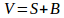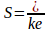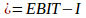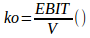# Find out How to Calculate Capitalization Rate

## Capitalization Rate: How to CalculateAssignment:

XYZ Ltd., expects a net income of Rs. 1,50,000. The company has 10% of
5,00,000 Debentures. The equity capitalization rate of the company is 10%.
(a) Calculate the value of the firm and overall capitalization rate according to
the net income approach (ignoring income tax).
(b) If the debenture debt is increased to Rs. 7,50,000 and interest of debt is change
to 9%. What is the value of the firm and overall capitalization rate?a. Calculation the firm value and overall capitalization rate according to the net income approach.

According to net income approach, the firm value is the summary its debt and equity. The cost of debt and cost of equity are independent. The main purpose of any company is raising its value using the most optimal mix of debt and equity. While the firm will achieve to maximize its value, the overall capitalization rate will be declined. So how to calculate capitalization rate? The overall capitalization rate means how much net operating income is generated by the dollar of market value its assets. And what is the value of the firm and how do business analysis and valuation work? The value of the company (V) is defined the following formulas, where S is the sum of market value of its equity and B is the market value of its debt.Using NI approach, the market value of equity(S) is calculated as earning available to equity shareholders (NI) divided by the equity capitalization rate (k e).The earnings available to equity shareholders (NI) are determined as net operating income (EBIT) less interest on debenture (I).The overall capitalization rate (ko) is defined as net operating income (EBIT) divided by the value of the company (V).Particulars Rs. Net operating income (EBIT) 1,50,000 Less: Interest of 10% on debenture of 5,00,000 (I) 50,000 Earnings available to equity shareholders (NI) 1,00,000 Divided by: Equity capitalization rate (K e)% 10% Market value of equity (S= Nix100/Ke) 10,00,000 Plus: Market value of debt (B) 5,00,000 Value of the firm (V=S+B) 15,00,000 Overall capitalization rate (Ko=EBIT/V)% 10.00%

So, the value of the firm will be equal Rs. 15, 00,000, while the overall capitalization rate will be the same as cost of equity and debt. Typically, the cost of debt is lower than cost of equity as the lenders require lower rate of return than equity shareholders. So, the company is more interested in attraction additional debt than equity for its operating activity. In the above case, the firm has as the equal finance structure, as the same cost of its sources. This is the main reason why the overall capitalization rate is the same.

b. Calculation the firm value and overall capitalization rate if the debt will be increased.

According to the above calculation in requirement #a, if the debenture debt is increased to Rs. 7, 50,000 while the cost of debt is reduced to 9%, the calculation the firms’ value will be following. As the cost of debt is decreased, but the proportion in capital structure ups the value of the company will be increased as shown in the table below. As the result of this shift in capital structure the overall capitalization rate is decreased from 10 percent to 9.52 percent.

 Particulars Rs Net operating income (EBIT) 1,50,000 Less: Interest of 9% on debenture of 7,50,000 (I) 67,500 Earnings available to equity shareholders (NI) 82,500 Divided by: Equity capitalization rate (K e)% 10% Market value of equity (S= Nix100/K e) 825,000 Plus: Market value of debt (B) 7,50,000 Value of the firm (V=S+B) 15,75,000 Overall capitalization rate (K o=EBIT/V)% 9.52%